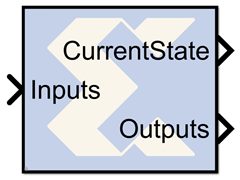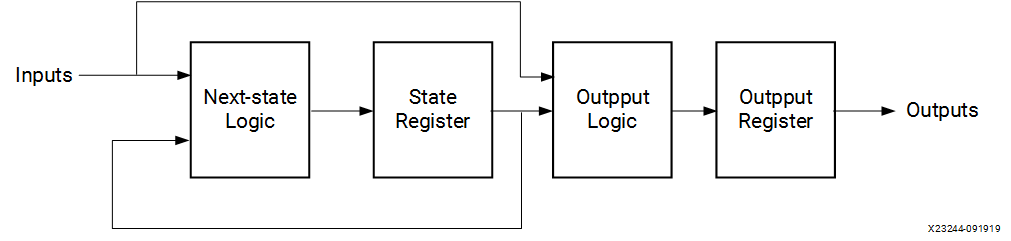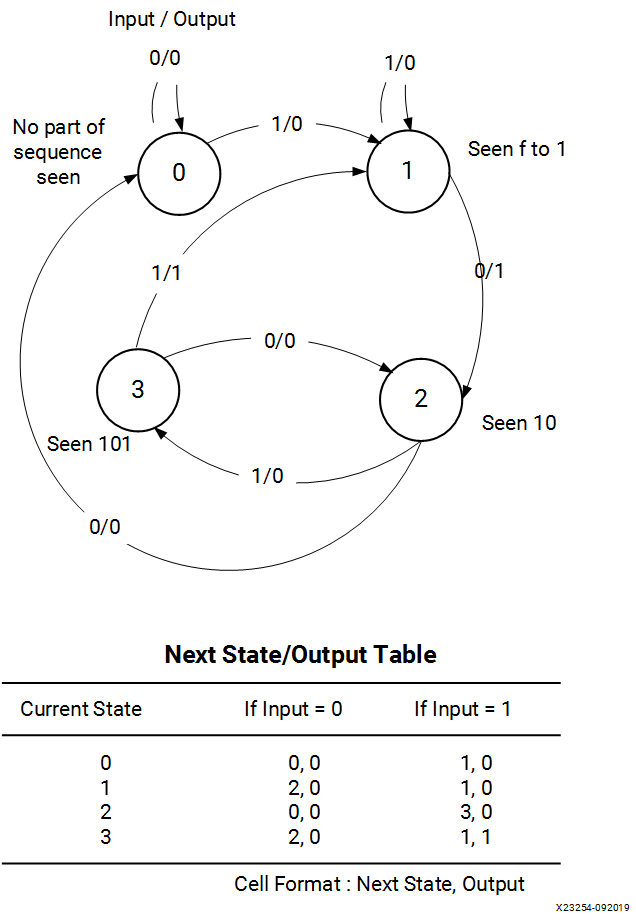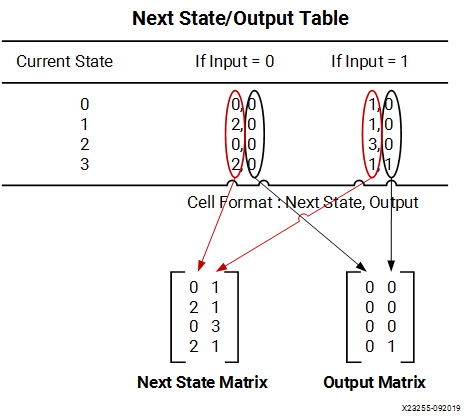# Registered Mealy State Machine - 2020.2 English

## Vivado Design Suite Reference Guide: Model-Based DSP Design Using System Generator (UG958)

Document ID
UG958
Release Date
2020-11-18
Version
2020.2 English

A "Mealy machine" is a finite state machine whose output is a function of state transition, for example, a function of the machine's current state and current input. A "registered Mealy machine" is one having registered output, and can be described with the following block diagram:Figure 1. Mealy State Machine Block DiagramThere are many ways to implement such state machines in System Generator (e.g., using the MCode block to implement the transition function, and registers to implement state variables). This reference block provides a method for implementing a Mealy machine using block and distributed memory. The implementation is very fast and efficient. For example, a state machine with 8 states, 1 input, and 2 outputs that are registered can be realized with a single block RAM that runs at more than 150 MHz in a Xilinx Virtex device.

The transition function and output mapping are each represented as an N x M matrix, where N is the number of states, and M is the size of the input alphabet (e.g., M = 2 for a binary input). It is convenient to number rows and columns from 0 to N - 1 and 0 to M - 1 respectively. Each state is represented as an unsigned integer from 0 to N - 1, and each alphabet character is represented as an unsigned integer from 0 to M - 1. The row index of each matrix represents the current state, and the column index represents the input character

For the purpose of discussion, let F be the N x M transition function matrix, and O be the N x M output function matrix. Then F(i,j) is the next state when the current state is i and the current input character is j, and O(i,j) is the corresponding output of the Mealy machine.

## Example

Consider the problem of designing a Mealy machine to recognize the pattern '1011' in a serial stream of bits. The state transition diagram and equivalent transition table are shown below.

Figure 2. Transition TableThe table lists the next state and output that result from the current state and input. For instance, if the current state is 3 and the input is 1, the next state is 1 and the output is 1, indicating the detection of the desired sequence.

The Registered Mealy State Machine block is configured with next state and output matrices obtained from the next state/output table discussed above. These matrices are constructed as shown below:

Figure 3. Next State Output TableRows of the matrices correspond to states, and columns correspond to input values.

## Block Parameters

The block parameters dialog box can be invoked by double-clicking the icon in your Simulink model.

The next state logic, state register, output logic, and output register are implemented using high speed dedicated block RAM. Of the four blocks in the state machine library, this is the fastest and most area efficient. However, the output is registered and thus the input does not affect the output instantaneously.

The number of bits used to implement a Mealy state machine is given by the equations:

depth = (2 k )(2 i ) = 2 k+i

width = k+o

N = depth*width = (k+o)(2 k+i )

where

N = total number of block RAM bits

s = number of states

k = ceil(log2(s))

i = number of input bits

o = number of output bits

The following table gives examples of block RAM sizes necessary for various state machines:

Table 1. State Machine block RAM Requirements
Number of States Number of Input Bits Number of Output Bits Block RAM Bits Needed
2 5 10 704
4 1 2 32
8 6 7 5120
16 5 4 4096
32 4 3 4096
52 1 11 2176
100 4 5 24576